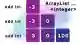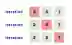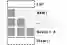# Java ArrayList int, Integer Examples

Use an ArrayList of Integer values to store int values. An ArrayList cannot store ints.

ArrayList, int. An ArrayList contains many elements. But in Java 8 it cannot store values. It can hold classes (like Integer) but not values (like int).Int notes. To place ints in ArrayList, we must convert them to Integers. This can be done in the add() method on ArrayList. Each int must added individually.

First example. Here we have an int array. It has 3 values in it. We create an ArrayList and add those ints as Integers in a for-loop.
Add: The add() method receives an Integer. And we can pass an int to this method—the int is cast to an Integer.
CastJava program that uses ArrayList of Integer values import java.util.ArrayList; public class Program { public static void main(String[] args) { int[] ids = {-3, 0, 100}; ArrayList<Integer> values = new ArrayList<>(); // Add all the ints as Integers with add. // ... The ints are cast to Integer implicitly. for (int id: ids) { values.add(id); } System.out.println(values); // The collections have the same lengths. System.out.println(values.size()); System.out.println(ids.length); } } Output [-3, 0, 100] 3 3

For-loop. We can use a simpler syntax to loop over elements—the for-each loop does not have an index variable. This improves readability. But it reduces flexibility in the loop.
Version 1: Use the for-each loop syntax, which omits the index from the Java code—this is simpler.
For
Version 2: Use the classic for-loop syntax, which includes the index variable, and loop up to size().Java program that loops over ArrayList import java.util.ArrayList; public class Program { public static void main(String[] args) { ArrayList<Integer> list = new ArrayList<>(); list.add(2); list.add(3); list.add(7); // Version 1: use for-each loop. for (int value : list) { System.out.println("FOR 1: " + value); } // Version 2: use for-loop. for (int i = 0; i < list.size(); i++) { System.out.println("FOR 2: " + list.get(i)); } } } Output FOR 1: 2 FOR 1: 3 FOR 1: 7 FOR 2: 2 FOR 2: 3 FOR 2: 7

Count, clear. The ArrayList is used in many programs. But in most places, it is used in a similar way: we instantiate, add elements, and access data.
Part 1: We create an ArrayList—diamond inference syntax is on the right side. We add 3 elements in statements.
Part 2: We call size(). This returns the number of elements in the collection (here it is 3).
Part 3: We call clear() to empty the ArrayList, and then print its size again. The ArrayList is empty.
ArrayList clearJava program that uses ArrayList import java.util.ArrayList; public class Program { public static void main(String[] args) { // Part 1: create new ArrayList. // ... Add 3 elements. ArrayList<Integer> elements = new ArrayList<>(); elements.add(10); elements.add(15); elements.add(20); // Part 2: get count by calling size. System.out.println("Count: " + elements.size()); // Part 3: clear and print size. elements.clear(); System.out.println("Count: " + elements.size()); } } Output Count: 3 Count: 0

IndexOf. We use the indexOf and lastIndexOf methods to find indexes. IndexOf searches from the beginning. LastIndexOf searches from the end.
Part 1: We create an ArrayList and add 5 elements. It contains 2 elements with the value 100.
Part 2: We search for values. IndexOf and lastIndexOf find different indexes because they search in different ways.
Part 3: We display the results. We see that when a value is not found, the special index -1 is returned.
Java program that uses indexOf, lastIndexOf import java.util.ArrayList; public class Program { public static void main(String[] args) { // Part 1: create ArrayList. ArrayList<Integer> list = new ArrayList<>(); list.add(101); list.add(100); list.add(99); list.add(100); list.add(99); // Part 2: search for values. int index = list.indexOf(100); int lastIndex = list.lastIndexOf(100); int notFound = list.indexOf(200); // Part 3: display results. System.out.println("INDEX: " + index); System.out.println("LASTINDEX: " + lastIndex); System.out.println("NOTFOUND: " + notFound); } } Output INDEX: 1 LASTINDEX: 3 NOTFOUND: -1

SubList. This returns a view of the ArrayList. We can loop over the returned List, or access its elements, using a zero-based index. So the first element in the sub-list is at index 0.
Argument 1: The first argument to subList() is the first index of the range we want to access from the ArrayList.
Argument 2: This is the last index (not a count) of the view we want to access from the source ArrayList.
Tip: If we set() an element in the sub-list to a value, this is reflected in the original list. The indexes are automatically translated.
Java program that uses subList import java.util.ArrayList; import java.util.List; public class Program { public static void main(String[] args) { // ... Add 5 integers to an ArrayList. ArrayList<Integer> list = new ArrayList<>(); list.add(5); list.add(10); list.add(15); list.add(20); list.add(25); // ... Get sub list from 1 to 3. List<Integer> sub = list.subList(1, 3); // ... Display sub list. for (int value : sub) { System.out.println(value); } // ... Set the first element in "sub" to -1. // This is reflected in the original ArrayList. sub.set(0, -1); System.out.println(list.get(1)); } } Output 10 15 -1

Collections.min, max. Many static methods in the Collections class can be used on an ArrayList. Here we use two simple ones: min and max.
Min: This scans the entire collection (an ArrayList) and returns the value of the smallest element.
Max: This returns the highest value in the collection. No loop syntax is needed.
Java program that uses Collections.min, max import java.util.ArrayList; import java.util.Collections; public class Program { public static void main(String[] args) { // Create ArrayList. ArrayList<Integer> list = new ArrayList<>(); list.add(10); list.add(1); list.add(100); list.add(5); // Min and max. int minimum = Collections.min(list); int maximum = Collections.max(list); System.out.println(minimum); System.out.println(maximum); } } Output 1 100

Collections.addAll. With this we add many elements to an ArrayList at once. The second argument is either an array of the elements to add, or those elements as arguments.Collections.addAll
Here: We add 3 elements from an array to an ArrayList. We then add 2 more elements with addAll.
Java program that uses Collections.addAll import java.util.ArrayList; import java.util.Collections; public class Program { public static void main(String[] args) { ArrayList<Integer> values = new ArrayList<>(); Integer[] array = { 10, 20, 30 }; // Add all elements in array to ArrayList. Collections.addAll(values, array); // Display. for (int value : values) { System.out.print(value); System.out.print(" "); } System.out.println(); // Add more elements. Collections.addAll(values, 40, 50); // Display. for (int value : values) { System.out.print(value); System.out.print(" "); } } } Output 10 20 30 10 20 30 40 50

ToArray. The toArray method copies an ArrayList's elements to an array. It has two versions—one returns an Object array and requires casting. This version, though, returns a typed array.
Tip: The toArray method will allocate a new array and return its reference unless it receives a large enough array.
So: We use toArray by passing it an empty array of the matching type. It then returns a correctly-sized array.
Java program that uses toArray import java.util.ArrayList; import java.util.List; public class Program { public static void main(String[] args) { ArrayList<Integer> list = new ArrayList<>(); list.add(7); list.add(8); list.add(9); // Create an empty array and pass to toArray. Integer[] array = {}; array = list.toArray(array); // Our array now has the ArrayList's elements. for (int elem : array) { System.out.println(elem); } } } Output 7 8 9

Compile error. We cannot specify int as the type of an ArrayList. An int is not a "ReferenceType." Instead we must use Integer—and add only Integers to this collection.
Java program that causes insert Dimensions error import java.util.ArrayList; public class Program { public static void main(String[] args) { // This does not compile. // ... Integer must be used, not int. ArrayList<int> test = new ArrayList<>(); } } Output Exception in thread "main" java.lang.Error: Unresolved compilation problem: Syntax error, insert "Dimensions" to complete ReferenceType at Program.main(Program.java:8)

AddAll error. We cannot pass an int array to the Collections.addAll method to add Integers to an ArrayList. An Integer array can be used.
Note: To add ints we need some extra steps to enable conversion from int to Integer. A for-loop with add() is effective.
Java program that causes unresolved compilation problem import java.util.ArrayList; import java.util.Collections; public class Program { public static void main(String[] args) { int[] ids = {-3, 0, 100}; ArrayList<Integer> values = new ArrayList<>(); // This does not compile. // ... Integer is not the same as int. Collections.addAll(values, ids); } } Output Exception in thread "main" java.lang.Error: Unresolved compilation problem: The method addAll(Collection<? super T>, T...) in the type Collections is not applicable for the arguments (ArrayList<Integer>, int[]) at Program.main(Program.java:11)

Notes, Integer. What is an Integer? And why cannot we use int in an ArrayList? An Integer is a reference type (a class). An int is a value.
And: The ArrayList requires, in its implementation, a reference type. So int is not allowed.

A summary. With the Integer class, we store ints in an ArrayList. We cannot use int directly. This introduces some complexity to our programs.

Home

© 2007-2020 sam allen. send bug reports to info@dotnetperls.com.